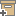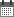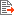Syntax
Search...
Manage
Community:
אסיף מאגר המחקר החקלאי
Soil hydraulic properties as stochastic processes: I. An analysis of field spatial variabilityBack to searchPrevious item
Next item
Year:
1981
Authors :
Russo, David
;
.
Volume :
45
Co-Authors:

Russo, D.,
E. Bresler

Facilitators :
From page:
682
To page:
687
(
Total pages:
6
)
Abstract:

Determinations of the sorptivity (5) and five parameters describing the hydraulic conductivity [K(h)\ and soil water retentivity [6(h)\ functions have been used to analyze the spatial distribution of the hydraulic properties in an experimental field. The parameters are: saturated hydraulic conductivity (Ks ); water entry value (HJ; saturated (0j) and residual (8r) water contents; and a constant 0 characterizing the pore size distribution of the soil. For a given depth, each of these parameters is described as a realization of a stationary two-dimensional isotropic and random process. These stochastic processes are characterized by truncated normal or log-normal probability density functions independent of the spatial position, and by autocorrelation functions between any two spatial points in the field which depend solely on the size of the vector separating the two points. The spatial variability of each of the six parameters has a structure that is characterized by a characteristic length—the integral scale, 7, representing the largest distance for which the parameter is correlated with itself. Values of J, which are calculated from the autocorrelation functions for each parameter generally decrease with depth. On the average over depth the calculated values of J are 21,44,55,25,35, and 15 meters for the parameters Ks , /!„,, Os , 6r , S, and (3, respectively. The spatial variability of the hydraulic functions are described by the probability density functions and the autocorrelation functions. Since the integral scales of K(h) and 6(h) vary with both h and depth, the characteristic length of the field has been chosen as the integral scale of the weighted mean diffusivity which is 18 m.

Note:
Related Files :
Autocorrelation functions
hydraulic conductivity
soil water retentivity
Related Content
More details
DOI :
Article number:
0
Affiliations:
Database:
Publication Type:
article
;
.
Language:
English
Editors' remarks:
ID:
55946
Last updated date:
02/03/2022 17:27
Creation date:
18/08/2021 15:39Scientific Publication
Soil hydraulic properties as stochastic processes: I. An analysis of field spatial variability
45

Russo, D.,
E. Bresler

Soil hydraulic properties as stochastic processes: I. An analysis of field spatial variability

Determinations of the sorptivity (5) and five parameters describing the hydraulic conductivity [K(h)\ and soil water retentivity [6(h)\ functions have been used to analyze the spatial distribution of the hydraulic properties in an experimental field. The parameters are: saturated hydraulic conductivity (Ks ); water entry value (HJ; saturated (0j) and residual (8r) water contents; and a constant 0 characterizing the pore size distribution of the soil. For a given depth, each of these parameters is described as a realization of a stationary two-dimensional isotropic and random process. These stochastic processes are characterized by truncated normal or log-normal probability density functions independent of the spatial position, and by autocorrelation functions between any two spatial points in the field which depend solely on the size of the vector separating the two points. The spatial variability of each of the six parameters has a structure that is characterized by a characteristic length—the integral scale, 7, representing the largest distance for which the parameter is correlated with itself. Values of J, which are calculated from the autocorrelation functions for each parameter generally decrease with depth. On the average over depth the calculated values of J are 21,44,55,25,35, and 15 meters for the parameters Ks , /!„,, Os , 6r , S, and (3, respectively. The spatial variability of the hydraulic functions are described by the probability density functions and the autocorrelation functions. Since the integral scales of K(h) and 6(h) vary with both h and depth, the characteristic length of the field has been chosen as the integral scale of the weighted mean diffusivity which is 18 m.

Scientific Publication
You may also be interested in